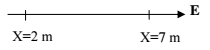# Problem: A uniform electric field, with a magnitude of 15 x10 2 N/C, is directed parallel to the positive x-axis. The work done by the electron as it moves from A (x = 2 m) to B (x = 7 m) is -3.2 x 10-16 J. If potential at A is +5000 V, what is the potential at B? (For an electron q = -1.6 x 10  -19 C) (a) -2800 V (b) +3000 V (c) +7000 V (d) -2000 V

###### FREE Expert Solution
84% (364 ratings)
###### Problem Details

A uniform electric field, with a magnitude of 15 x10 2 N/C, is directed parallel to the positive x-axis. The work done by the electron as it moves from A (x = 2 m) to B (x = 7 m) is -3.2 x 10-16 J. If potential at A is +5000 V, what is the potential at B? (For an electron q = -1.6 x 10  -19 C)

(a) -2800 V

(b) +3000 V

(c) +7000 V

(d) -2000 V